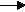gcsescience.com                                       29                                       gcsescience.com

Moles

Concentrations of Solutions.

Example 2.

In a titration, 20 cm3 of 0·5 mol/dm3 sodium hydroxide
is exactly neutralised by 40
cm3 of sulfuric acid.
What is the concentration of the sulfuric acid?

Method.
1) Write the equation for the reaction.

sodium hydroxide + sulfuric acidsodium sulfate  +  water.
2NaOH(aq)    +    H2SO4(aq)Na2SO4(aq)   +  2H2O(l)

Use the big numbers to find the proportion of NaOH to H2SO4.
Two moles of NaOH react with one mole of H2SO4.

2) Find how many moles of sodium hydroxide are present.
moles = (C x V) ÷ 1000.

The number of moles in 20 cm3 of 0·5 mol/dm3 sodium hydroxide
= (0
·5 x 20) ÷ 1000
=
0·01 moles of NaOH.

3) From 1, the proportion of NaOH to H2SO4 is 2 to 1.

0·01 moles of NaOH react with (0·01 ÷ 2) moles of H2SO4
=
0·005 moles H2SO4.
So, there are
0·005 moles of H2SO4 in 40 cm3.

C = (moles x 1000) ÷ V

= (0·005 x 1000) ÷ 40
= 0·125.

The concentration of sulfuric acid is 0·125 mol/dm3.

gcsescience.com       The Periodic Table       Index       Moles Quiz       gcsescience.com